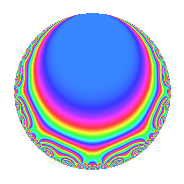Properties

 Label 4004.2.a.dLevel 4004 Weight 2 Character orbit 4004.a Self dual Yes Analytic conductor 31.972 Analytic rank 1 Dimension 4 CM No Inner twists 1

Related objects

Newspace parameters

 Level: $$N$$ = $$4004 = 2^{2} \cdot 7 \cdot 11 \cdot 13$$ Weight: $$k$$ = $$2$$ Character orbit: $$[\chi]$$ = 4004.a (trivial)

Newform invariants

 Self dual: Yes Analytic conductor: $$31.9721009693$$ Analytic rank: $$1$$ Dimension: $$4$$ Coefficient field: 4.4.3981.1 Coefficient ring: $$\Z[a_1, a_2, a_3]$$ Coefficient ring index: $$1$$ Fricke sign: $$1$$ Sato-Tate group: $\mathrm{SU}(2)$

$q$-expansion

Coefficients of the $$q$$-expansion are expressed in terms of a basis $$1,\beta_1,\beta_2,\beta_3$$ for the coefficient ring described below. We also show the integral $$q$$-expansion of the trace form.

 $$f(q)$$ $$=$$ $$q$$ $$+ ( -1 - \beta_{3} ) q^{3}$$ $$+ ( \beta_{2} + \beta_{3} ) q^{5}$$ $$+ q^{7}$$ $$+ ( 1 - \beta_{1} + 2 \beta_{3} ) q^{9}$$ $$+O(q^{10})$$ $$q$$ $$+ ( -1 - \beta_{3} ) q^{3}$$ $$+ ( \beta_{2} + \beta_{3} ) q^{5}$$ $$+ q^{7}$$ $$+ ( 1 - \beta_{1} + 2 \beta_{3} ) q^{9}$$ $$+ q^{11}$$ $$+ q^{13}$$ $$+ ( -1 - \beta_{1} ) q^{15}$$ $$+ ( -2 - \beta_{2} - \beta_{3} ) q^{17}$$ $$+ ( -2 - \beta_{1} - \beta_{2} ) q^{19}$$ $$+ ( -1 - \beta_{3} ) q^{21}$$ $$+ ( 3 \beta_{1} - \beta_{2} ) q^{23}$$ $$+ ( -2 + 2 \beta_{1} - 2 \beta_{2} - 3 \beta_{3} ) q^{25}$$ $$+ ( -3 + 3 \beta_{1} + \beta_{2} ) q^{27}$$ $$+ ( -2 + 3 \beta_{1} - 2 \beta_{3} ) q^{29}$$ $$+ ( -1 + 2 \beta_{1} - 2 \beta_{3} ) q^{31}$$ $$+ ( -1 - \beta_{3} ) q^{33}$$ $$+ ( \beta_{2} + \beta_{3} ) q^{35}$$ $$+ ( -3 - 3 \beta_{1} + 3 \beta_{3} ) q^{37}$$ $$+ ( -1 - \beta_{3} ) q^{39}$$ $$+ ( -3 - 2 \beta_{1} - 3 \beta_{2} ) q^{41}$$ $$+ ( 1 - 3 \beta_{1} - 2 \beta_{2} + 2 \beta_{3} ) q^{43}$$ $$+ ( 2 + \beta_{1} - 2 \beta_{2} - 2 \beta_{3} ) q^{45}$$ $$+ ( -3 \beta_{1} - 3 \beta_{2} ) q^{47}$$ $$+ q^{49}$$ $$+ ( 3 + \beta_{1} + 2 \beta_{3} ) q^{51}$$ $$+ ( \beta_{1} + \beta_{2} - 3 \beta_{3} ) q^{53}$$ $$+ ( \beta_{2} + \beta_{3} ) q^{55}$$ $$+ ( 1 + 3 \beta_{1} + \beta_{2} + \beta_{3} ) q^{57}$$ $$+ ( -1 - \beta_{1} + 2 \beta_{2} + 2 \beta_{3} ) q^{59}$$ $$+ ( -4 + 3 \beta_{1} + 4 \beta_{2} + \beta_{3} ) q^{61}$$ $$+ ( 1 - \beta_{1} + 2 \beta_{3} ) q^{63}$$ $$+ ( \beta_{2} + \beta_{3} ) q^{65}$$ $$+ ( 1 - 2 \beta_{1} + 2 \beta_{2} + 2 \beta_{3} ) q^{67}$$ $$+ ( -5 - \beta_{1} - 3 \beta_{2} - \beta_{3} ) q^{69}$$ $$+ ( 2 - 4 \beta_{1} + 4 \beta_{3} ) q^{71}$$ $$+ ( -4 + 7 \beta_{1} + 2 \beta_{2} - 3 \beta_{3} ) q^{73}$$ $$+ ( 5 - \beta_{1} - 2 \beta_{2} + 3 \beta_{3} ) q^{75}$$ $$+ q^{77}$$ $$+ ( 3 - \beta_{2} + 5 \beta_{3} ) q^{79}$$ $$+ ( -1 - 2 \beta_{1} - 3 \beta_{2} - 2 \beta_{3} ) q^{81}$$ $$+ ( 4 - 7 \beta_{1} + \beta_{3} ) q^{83}$$ $$+ ( -3 - 2 \beta_{1} + \beta_{3} ) q^{85}$$ $$+ ( 5 - 5 \beta_{1} - 3 \beta_{2} + 4 \beta_{3} ) q^{87}$$ $$+ ( 4 - 5 \beta_{1} + 3 \beta_{2} + 2 \beta_{3} ) q^{89}$$ $$+ q^{91}$$ $$+ ( 5 - 4 \beta_{1} - 2 \beta_{2} + 3 \beta_{3} ) q^{93}$$ $$+ ( -2 - 2 \beta_{1} - 2 \beta_{2} - \beta_{3} ) q^{95}$$ $$+ ( -4 - 2 \beta_{1} - \beta_{2} - 2 \beta_{3} ) q^{97}$$ $$+ ( 1 - \beta_{1} + 2 \beta_{3} ) q^{99}$$ $$+O(q^{100})$$ $$\operatorname{Tr}(f)(q)$$ $$=$$ $$4q$$ $$\mathstrut -\mathstrut 3q^{3}$$ $$\mathstrut -\mathstrut q^{5}$$ $$\mathstrut +\mathstrut 4q^{7}$$ $$\mathstrut +\mathstrut q^{9}$$ $$\mathstrut +\mathstrut O(q^{10})$$ $$4q$$ $$\mathstrut -\mathstrut 3q^{3}$$ $$\mathstrut -\mathstrut q^{5}$$ $$\mathstrut +\mathstrut 4q^{7}$$ $$\mathstrut +\mathstrut q^{9}$$ $$\mathstrut +\mathstrut 4q^{11}$$ $$\mathstrut +\mathstrut 4q^{13}$$ $$\mathstrut -\mathstrut 5q^{15}$$ $$\mathstrut -\mathstrut 7q^{17}$$ $$\mathstrut -\mathstrut 9q^{19}$$ $$\mathstrut -\mathstrut 3q^{21}$$ $$\mathstrut +\mathstrut 3q^{23}$$ $$\mathstrut -\mathstrut 3q^{25}$$ $$\mathstrut -\mathstrut 9q^{27}$$ $$\mathstrut -\mathstrut 3q^{29}$$ $$\mathstrut -\mathstrut 3q^{33}$$ $$\mathstrut -\mathstrut q^{35}$$ $$\mathstrut -\mathstrut 18q^{37}$$ $$\mathstrut -\mathstrut 3q^{39}$$ $$\mathstrut -\mathstrut 14q^{41}$$ $$\mathstrut -\mathstrut q^{43}$$ $$\mathstrut +\mathstrut 11q^{45}$$ $$\mathstrut -\mathstrut 3q^{47}$$ $$\mathstrut +\mathstrut 4q^{49}$$ $$\mathstrut +\mathstrut 11q^{51}$$ $$\mathstrut +\mathstrut 4q^{53}$$ $$\mathstrut -\mathstrut q^{55}$$ $$\mathstrut +\mathstrut 6q^{57}$$ $$\mathstrut -\mathstrut 7q^{59}$$ $$\mathstrut -\mathstrut 14q^{61}$$ $$\mathstrut +\mathstrut q^{63}$$ $$\mathstrut -\mathstrut q^{65}$$ $$\mathstrut -\mathstrut 20q^{69}$$ $$\mathstrut -\mathstrut 6q^{73}$$ $$\mathstrut +\mathstrut 16q^{75}$$ $$\mathstrut +\mathstrut 4q^{77}$$ $$\mathstrut +\mathstrut 7q^{79}$$ $$\mathstrut -\mathstrut 4q^{81}$$ $$\mathstrut +\mathstrut 8q^{83}$$ $$\mathstrut -\mathstrut 15q^{85}$$ $$\mathstrut +\mathstrut 11q^{87}$$ $$\mathstrut +\mathstrut 9q^{89}$$ $$\mathstrut +\mathstrut 4q^{91}$$ $$\mathstrut +\mathstrut 13q^{93}$$ $$\mathstrut -\mathstrut 9q^{95}$$ $$\mathstrut -\mathstrut 16q^{97}$$ $$\mathstrut +\mathstrut q^{99}$$ $$\mathstrut +\mathstrut O(q^{100})$$

Basis of coefficient ring in terms of a root $$\nu$$ of $$x^{4}\mathstrut -\mathstrut$$ $$x^{3}\mathstrut -\mathstrut$$ $$4$$ $$x^{2}\mathstrut +\mathstrut$$ $$2$$ $$x\mathstrut +\mathstrut$$ $$1$$:

 $$\beta_{0}$$ $$=$$ $$1$$ $$\beta_{1}$$ $$=$$ $$\nu$$ $$\beta_{2}$$ $$=$$ $$\nu^{2} - \nu - 2$$ $$\beta_{3}$$ $$=$$ $$\nu^{3} - \nu^{2} - 3 \nu + 1$$
 $$1$$ $$=$$ $$\beta_0$$ $$\nu$$ $$=$$ $$\beta_{1}$$ $$\nu^{2}$$ $$=$$ $$\beta_{2}\mathstrut +\mathstrut$$ $$\beta_{1}\mathstrut +\mathstrut$$ $$2$$ $$\nu^{3}$$ $$=$$ $$\beta_{3}\mathstrut +\mathstrut$$ $$\beta_{2}\mathstrut +\mathstrut$$ $$4$$ $$\beta_{1}\mathstrut +\mathstrut$$ $$1$$

Embeddings

For each embedding $$\iota_m$$ of the coefficient field, the values $$\iota_m(a_n)$$ are shown below.

For more information on an embedded modular form you can click on its label.

Label $$\iota_m(\nu)$$ $$a_{2}$$ $$a_{3}$$ $$a_{4}$$ $$a_{5}$$ $$a_{6}$$ $$a_{7}$$ $$a_{8}$$ $$a_{9}$$ $$a_{10}$$
1.1
 −0.318459 2.28400 0.785261 −1.75080
0 −2.82166 0 0.241539 0 1.00000 0 4.96179 0
1.2 0 −1.84617 0 1.77882 0 1.00000 0 0.408340 0
1.3 0 0.488200 0 −3.65683 0 1.00000 0 −2.76166 0
1.4 0 1.17963 0 0.636469 0 1.00000 0 −1.60847 0
 $$n$$: e.g. 2-40 or 990-1000 Significant digits: Format: Complex embeddings Normalized embeddings Satake parameters Satake angles

Inner twists

This newform does not admit any (nontrivial) inner twists.

Atkin-Lehner signs

$$p$$ Sign
$$2$$ $$-1$$
$$7$$ $$-1$$
$$11$$ $$-1$$
$$13$$ $$-1$$

Hecke kernels

This newform can be constructed as the kernel of the linear operator $$T_{3}^{4}$$ $$\mathstrut +\mathstrut 3 T_{3}^{3}$$ $$\mathstrut -\mathstrut 2 T_{3}^{2}$$ $$\mathstrut -\mathstrut 6 T_{3}$$ $$\mathstrut +\mathstrut 3$$ acting on $$S_{2}^{\mathrm{new}}(\Gamma_0(4004))$$.